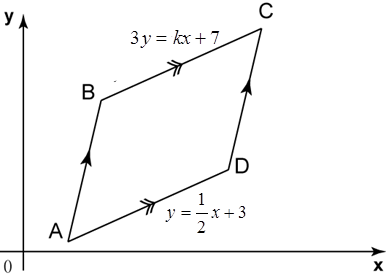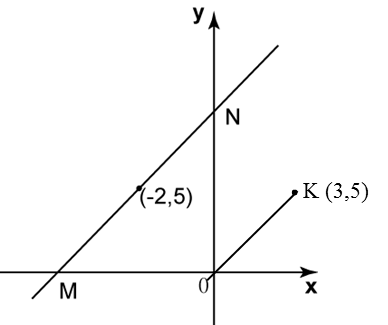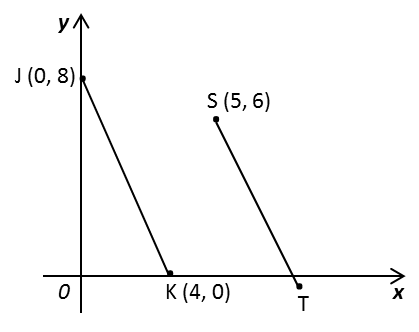# 5.7.1 The Straight Line, SPM Paper 2

Question 1:
In diagram below, ABCD is a trapezium drawn on a Cartesian plane. BC is parallel to AD and O is the origin. The equation of the straight line BC is 3y = kx+ 7 and the equation of the straight line AD is $y=\frac{1}{2}x+3$Find
(a) the value of k,
(b) the x-intercept of the straight line BC.

Solution:

(a)
Equation of BC :
3y = kx + 7

$\begin{array}{l}\frac{k}{3}=\frac{1}{2}\\ \therefore k=\frac{3}{2}\end{array}$

(b)

Equation of BC$3y=\frac{3}{2}x+7$
For x-intercept, y = 0
$\begin{array}{l}3\left(0\right)=\frac{3}{2}x+7\\ \frac{3}{2}x=-7\\ x=-\frac{14}{3}\end{array}$
Therefore x-intercept of BC $-\frac{14}{3}$

Question 2:
In diagram below, is the origin. Straight line MN is parallel to a straight line OK.Find
(a) the equation of the straight line MN,
(b) the x-intercept of the straight line MN.

Solution:

(a)
$\begin{array}{l}=\frac{5-0}{3-0}\\ =\frac{5}{3}\end{array}$

Substitute m = 5/3 and (–2, 5) into y = mx + c
$5=\frac{5}{3}\left(-2\right)+c$
15 = – 10 + 3c
3c = 25
c = 25/3

Therefore equation of MN$y=\frac{5}{3}x+\frac{25}{3}$

(b)
For x-intercept, y = 0
$\begin{array}{l}0=\frac{5}{3}x+\frac{25}{3}\\ \frac{5}{3}x=-\frac{25}{3}\end{array}$
5x = –25
x = –5
Therefore x-intercept of MN = –5

Question 3:
Diagram below shows a straight line JK and a straight line ST drawn on a Cartesian plane. JK is parallel to ST.Find
(a) the equation of the straight ST,
(b) the x-intercept of the straight line ST.

Solution:
(a)
JK is parallel to ST, therefore gradient of JK = gradient of ST.
$\begin{array}{l}=\frac{8-0}{0-4}\\ =-2\end{array}$
Substitute m = –2 and S (5, 6) into y = mx + c
6 = –2 (5) + c
c = 16
Therefore equation of ST: y = –2x + 16

(b)

For x-intercept, y = 0
0 = –2x + 16
2x = 16
x = 8
Therefore x-intercept of ST = 8

### 1 thought on “5.7.1 The Straight Line, SPM Paper 2”

1.Excuse me? I wanna ask why did c become 3c in question 2 b?